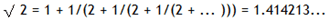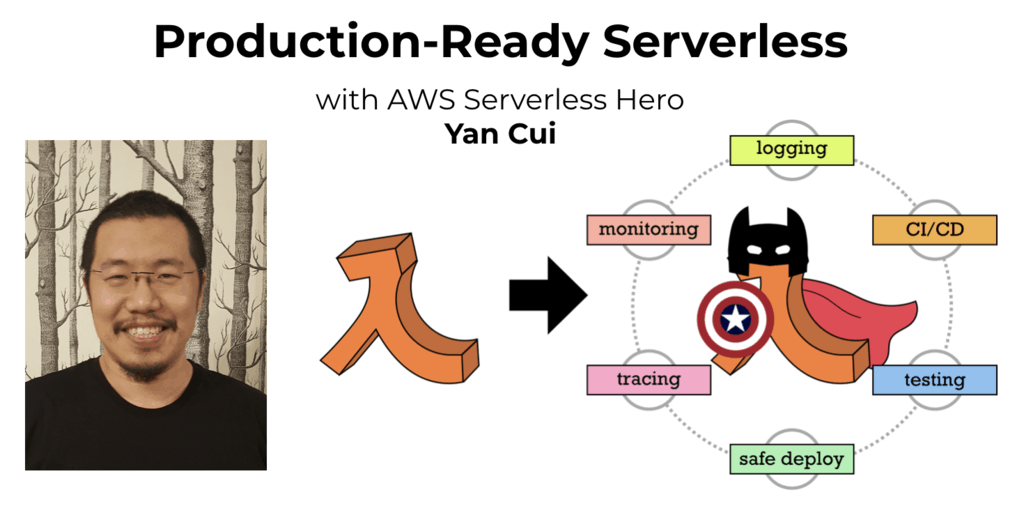# Project Euler – Problem 57 Solution

Check out my new course Learn you some Lambda best practice for great good! and learn the best practices for performance, cost, security, resilience, observability and scalability.

#### Problem

It is possible to show that the square root of two can be expressed as an infinite continued fraction.By expanding this for the first four iterations, we get:

1 + 1/2 = 3/2 = 1.5

1 + 1/(2 + 1/2) = 7/5 = 1.4

1 + 1/(2 + 1/(2 + 1/2)) = 17/12 = 1.41666…

1 + 1/(2 + 1/(2 + 1/(2 + 1/2))) = 41/29 = 1.41379…

The next three expansions are 99/70, 239/169, and 577/408, but the eighth expansion, 1393/985, is the first example where the number of digits in the numerator exceeds the number of digits in the denominator.

In the first one-thousand expansions, how many fractions contain a numerator with more digits than denominator?

#### Solution

```// define function to return all the numerator-denominator pairs for the first n expand
let expand n =
Seq.unfold (fun (num, denom) -> Some((num, denom), (denom*2I+num, denom+num))) (3I, 2I)
|> Seq.take n

expand 1000
|> Seq.filter (fun (num, denom) -> num.ToString().Length > denom.ToString().Length)
|> Seq.length
```

If you look at the patterns 3/2, 7/5, 17/12, 41/29, and so on, it’s easy to spot a pattern where the numerator and denominator of iteration n can be derived from the iteration n-1:

Numerator(n) = Numerator(n-1) + 2 * Denominator(n-1)

Denominator(n) = Numerator(n-1) + Denominator(n-1)Hi, I’m Yan. I’m an AWS Serverless Hero and the author of Production-Ready Serverless.

I specialise in rapidly transitioning teams to serverless and building production-ready services on AWS.

Are you struggling with serverless or need guidance on best practices? Do you want someone to review your architecture and help you avoid costly mistakes down the line? Whatever the case, I’m here to help.Check out my new course, Learn you some Lambda best practice for great good! In this course, you will learn best practices for working with AWS Lambda in terms of performance, cost, security, scalability, resilience and observability. Enrol now and enjoy a special preorder price of £9.99 (~\$13).Are you working with Serverless and looking for expert training to level-up your skills? Or are you looking for a solid foundation to start from? Look no further, register for my Production-Ready Serverless workshop to learn how to build production-grade Serverless applications!